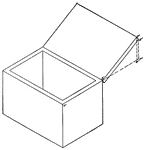### Isometric of a Box With a Cover

Isometric of a box with a cover.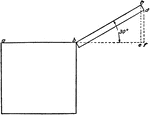### Isometric of a Box With a Cover

Isometric of a box with a cover - end view.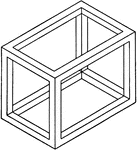### Isometric Of A Box

Illustration of the isometric of a skeleton of a box.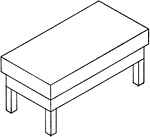### Isometric Of A Carpenter's Bench

Illustration of the isometric of a carpenter's bench.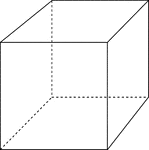### Cube

Illustration of 3-dimensional cube with hidden edges shown.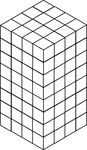### 128 Stacked Congruent Cubes

Illustration of 128 congruent cubes stacked so they form a rectangular solid that measures 4 by 4 by…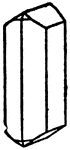### Combination of Prisms

An illustration of a combination formed by different prisms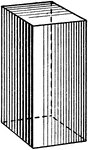### Square Prism

Illustration of a square prism; a prism whose bases are squares.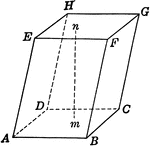### Prism With Square Bases

Prism with square bases.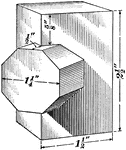### Intersecting Prisms

Illustration of the intersection of a quadrangular prism and an octagonal prism. The figures intersect…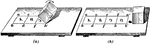### Development Of Rectangular Prism

Illustration of the development of a rectangular prism. "Assume now that the prism is laid on the drawing…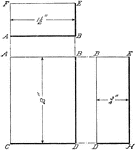### Projection Of Rectangular Prism

Illustration of the projection of a rectangular prism that is represented as if it were standing on…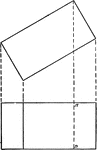### Projections of Rectangular Prism

Projections of a rectangular prism or block.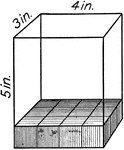### Volume Of A Rectangular Solid

Illustration of a 5 in. by 3 in. by 4 in. rectangular solid with each cube in the solid representing…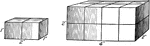### Similar Rectangular Solids

Illustration of a 1 in. by 2 in. by 1 in. rectangular solid that is similar to a 2 in. by 4 in. by 2…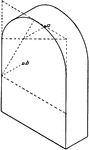### Prism With Semicircular Top

Isometric of a prism with a semicircular top.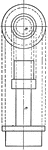### Projection of Turned Block

Projection of a turned block.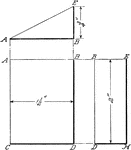### Projection Of Wedge

Illustration of the projection of a wedge of a rectangular prism that is standing on one of its triangular…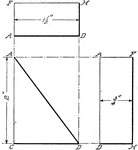### Projection Of Wedge

Illustration of the projection of a wedge of a rectangular prism that is standing on one of its rectangular…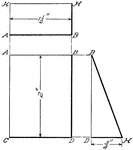### Projection Of Wedge

Illustration of the projection of a wedge of a rectangular prism that is viewed from the side.